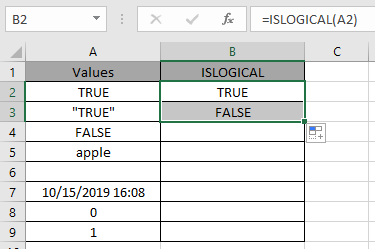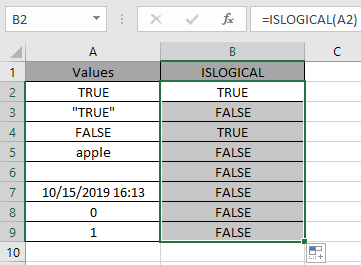# How to use the ISLOGICAL Function in Microsoft Excel

There are 2 logical values in excel

• TRUE &
• FALSE

ISLOGICAL function returns TRUE if the value is either TRUE or FALSE or else it returns FALSE. The logical values are usually returned by the logic function like IF, IFERROR, IFNA, etc functions.

ISLOGICAL function checks the value cell for a LOGICAL and returns TRUE if exist or else FALSE.

Syntax:

 =ISLOGICAL ( value )

value : value to the function can be given as directly or using cell reference

Now let’s understand the function more via using it in an example.Here we have a list of value in Column A & we need to check the cells for the LOGICAL values.

Use the LOGICAL:

 = ISLOGICAL ( A2 )

A2 : reference cell is provided to the function to check its value.And now the result of A3 cell.You can observe from the above snapshots that there is a difference between the LOGIC value in quotes and the one without quotes.

Copy the formula to other cells using the Ctrl + D shortcut key.As you can see, the function returns the logic values either TRUE or FALSE. Below are some of the observational results.
Note:
When range of cells given in as argument, the function only checks the first cell of the array as returns TRUE or FALSE based only on the first cell.

Hope you understood how to use ISLOGICAL function in Excel 2016. Find more articles on Excel ISLOGICAL function here. Please share your query below in the comment box. We will assist you.

Terms and Conditions of use

The applications/code on this site are distributed as is and without warranties or liability. In no event shall the owner of the copyrights, or the authors of the applications/code be liable for any loss of profit, any problems or any damage resulting from the use or evaluation of the applications/code.